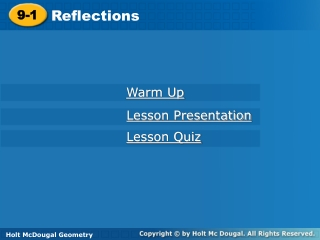DownloadDownload Presentation9-1

# 9-1

Download Presentation## 9-1

- - - - - - - - - - - - - - - - - - - - - - - - - - - E N D - - - - - - - - - - - - - - - - - - - - - - - - - - -
##### Presentation Transcript

1. Reflections 9-1 Warm Up Lesson Presentation Lesson Quiz Holt McDougal Geometry Holt Geometry

2. Warm Up Given that ∆ABC  ∆DEF, identify a segment or angle congruent to each of the following. 1. 2. 3. 4. 5. 6.

3. Objective Identify and draw reflections.

4. Vocabulary isometry

5. COPY THIS SLIDE: An isometry is a transformation that does not change the shape or size of a figure. Reflections, translations, and rotations are all isometries. Isometries are also called congruence transformations or rigid motions. A reflection is a transformation that moves a figure (called the preimage) by flipping it across a line. The reflected figure is called the image. A reflection is an isometry, so the image is always congruent to the preimage.

6. Example 1: Identifying Reflections COPY THIS SLIDE: Tell whether each transformation appears to be a reflection. Explain. B. A. No; the image does not Appear to be flipped. Yes; the image appears to be flipped across a line..

7. Check It Out! Example 1 Tell whether each transformation appears to be a reflection. a. b. Yes; the image appears to be flipped across a line. No; the figure does not appear to be flipped.

8. COPY THIS SLIDE:

9. COPY THIS SLIDE: Change the sign of the “y” Change the sign of the “x” Switch the coordinates ACROSS THE ORIGIN (x,y)(-x,-y) Change the signs of both

10. X(2,–1) X’(2, 1) Y(–4,–3) Y’(–4, 3) Z(3, 2) Z’(3, –2) Example 4A: Drawing Reflections in the Coordinate Plane COPY THIS SLIDE: Reflect the figure with the given vertices across the given line. X(2, –1), Y(–4, –3), Z(3, 2); x-axis The reflection of (x, y) is (x,–y). Y’ Z X’ X Z’ Y Graph the image and preimage.

11. S’ T’ R S R(–2, 2) R’(2, –2) T R’ S(5, 0) S’(0, 5) T(3, –1) T’(–1, 3) Example 4B: Drawing Reflections in the Coordinate Plane COPY THIS SLIDE: Reflect the figure with the given vertices across the given line. R(–2, 2), S(5, 0), T(3, –1); y = x The reflection of (x, y) is (y, x). Graph the image and preimage.

12. S V U T S(3, 4) S’(3, –4) T’ U’ T(3, 1) T’(3, –1) U(–2, 1) U’(–2, –1) S’ V’ V(–2, 4) V’(–2, –4) Check It Out! Example 4 Reflect the rectangle with vertices S(3, 4), T(3, 1), U(–2, 1) and V(–2, 4) across the x-axis. The reflection of (x, y) is (x,–y). Graph the image and preimage.

13. Examples: COPY THIS SLIDE: Reflect the figure with the given vertices across the given line. 3.A(2, 3), B(–1, 5), C(4,–1); y = x A’(3, 2), B’(5,–1), C’(–1, 4) 4.U(–8, 2), V(–3, –1), W(3, 3); y-axis U’(8, 2), V’(3, –1), W’(–3, 3) 5.E(–3, –2), F(6, –4), G(–2, 1); x-axis E’(–3, 2), F’(6, 4), G’(–2, –1)

14. Classwork/Homework • 9.1 W/S